×
Get Full Access to Introductory Chemistry - 5 Edition - Chapter 14 - Problem 32p
Get Full Access to Introductory Chemistry - 5 Edition - Chapter 14 - Problem 32p

×

# For each reaction, identify the Bransted-Lowry add, the Bransted-Lowry base, theISBN: 9780321910295 34

## Solution for problem 32P Chapter 14

Introductory Chemistry | 5th Edition

• Textbook Solutions
• 2901 Step-by-step solutions solved by professors and subject experts
• Get 24/7 help from StudySoup virtual teaching assistantsIntroductory Chemistry | 5th Edition

4 5 1 245 Reviews
29
3
Problem 32P

For each reaction, identify the Brønsted–Lowry acid, the Brønsted–Lowry base, the conjugate acid, and the conjugate base.

(a) $$\mathrm{HI}(a q)+\mathrm{H}_{2} \mathrm{O}(l) \rightarrow \mathrm{H}_{3} \mathrm{O}^{+}(a q)+l^{-}(a q)$$

(b) $$\mathrm{CH}_{3} \mathrm{NH}_{2}(a q)+\mathrm{H}_{2} \mathrm{O}(l) \rightleftharpoons \mathrm{CH}_{3} \mathrm{NH}_{3}^{+}(a q)+\mathrm{OH}^{-}(a q)$$

(c) $$\mathrm{CO}_{3}^{2-}(a q)+\mathrm{H}_{2} \mathrm{O}(l) \rightleftharpoons \mathrm{HCO}_{3}^{-}(a q)+\mathrm{OH}^{-}(a q)$$

.

(d) $$\mathrm{H}_{2} \mathrm{CO}_{3}(a q)+\mathrm{H}_{2} \mathrm{O}(l) \rightleftharpoons \mathrm{H}_{3} \mathrm{O}^{+}(a q)+\mathrm{HCO}_{3}^{-}(a q)$$

Equation Transcription:⇌⇌.⇌Text Transcription:

HI(aq)+H_2O(l) rightarrow H_3O^+(aq)+l^-(aq)

CH_3NH_2(aq)+H_2O(l) ⇌ CH_3NH_3+(aq)+OH^-(aq)

CO_3^2-(aq)+H_2O(l) ⇌ HCO_3^-(aq)+OH^-(aq)

.

H_2CO_3(aq)+H_2O(l) ⇌  H_3O^+(aq)+HCO_3^-(aq)

Step-by-Step Solution:

Problem 32P

For each reaction, identify the Bransted-Lowry add, the Bransted-Lowry base, the conjugate acid, and the conjugate base.

(a)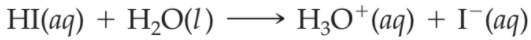(b)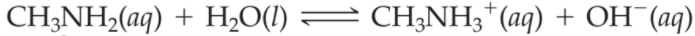(c)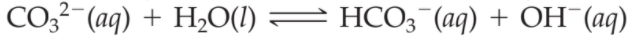(d)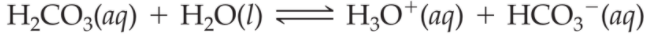Step by Step Solution

Step 1 of 4

Bronsted-lowry definition of acid-base is as it applies to a wider range of acid-base phenomena. This definition focuses on the transfer of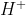ions in an acid-base reaction. therefore , according to bronsted-lowry an acid is a proton donor () and a base is a proton acceptor(). The substance that results after acid donates a proton is the conjugate base of that acid and the substance that results after the base accepts a proton is conjugate acid of that base.

(a).

The given reaction is as follows: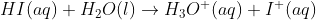Now will identify the Bronsted-lowry acid, Bronsted-lowry base, conjugate acid and conjugate base in the given equation.

Bronsted-lowry acid =Bronsted-lowry base =Conjugate base =Conjugate acid =Step 2 of 4

Step 3 of 4

##### ISBN: 9780321910295

Unlock Textbook Solution